### Home > MC2 > Chapter 7 > Lesson 7.2.1 > Problem7-67

7-67.
1. For each equation below, solve for x. Show the process you use to check your answer. Homework Help ✎

1.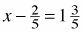2.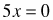3.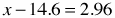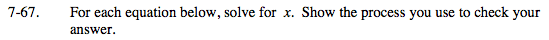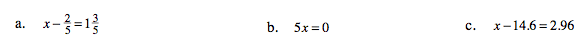Isolate the variable and constants.

$x-\frac{2}{5}=1\frac{3}{5}$

$\;\;\;+\frac{2}{5}\;\;\;+\frac{2}{5}$

$\textit{x}=\frac{8}{5}+\frac{2}{5}$

x = 2